##### How to Use POWER() Function in PostgreSQL

PostgreSQL offers a wide range of mathematical functions to work with numeric data, such as ABS(), MOD(), ROUND(), etc. The POWER() function is one of the mathematical functions used to calculate a number's power. In Postgres, the POWER() function can also be used as POW().

This write-up will teach you the basic syntax, working, and implementation of the Postgres POWER() function. So, let’s begin!

How to Use the POWER() Function in PostgreSQL?

The POWER() or POW() function accepts two numeric values as arguments and retrieves the first value/argument raised to the power of the second value/argument:

`POWER(val_1, val_2);`

val_1 and val_2 represent any numeric/double precision values.

Example 1: Find the Square of a Value Using the POWER() Function

The following example will show you how the POWER() function works in Postgres:

`SELECT POWER(12, 2);`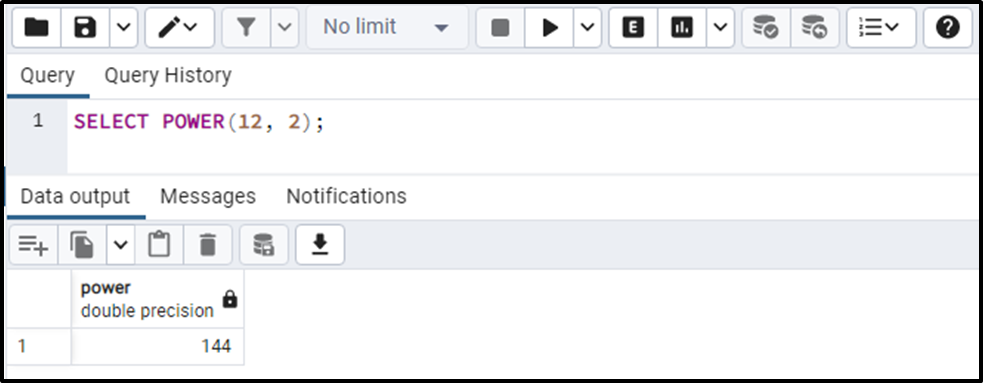The output snippet proves that the POWER() function retrieves the accurate result (i.e., 12 * 12 = 144).

Example 2: Find the Cube of a Number Using the POWER() Function

Let’s learn how to find the cube of a number using the Postgres POWER() function:

`SELECT POWER(5, 3);`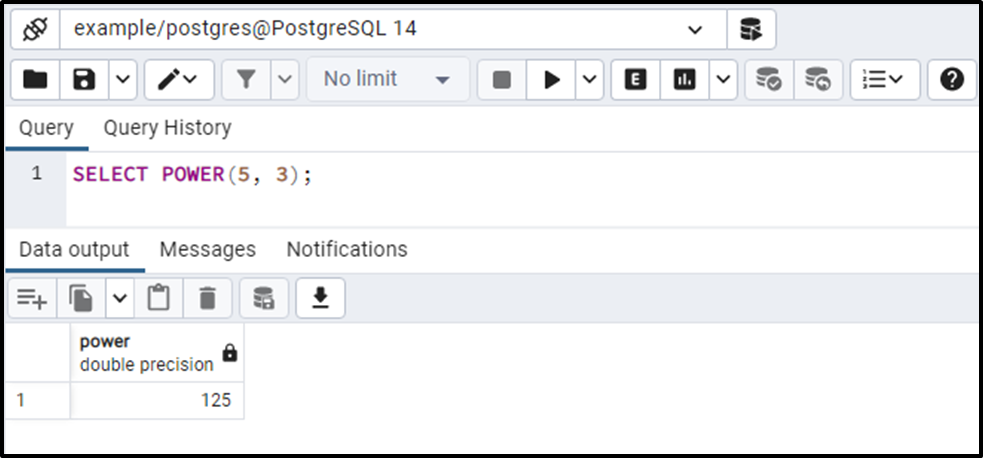The output snippet shows that the POWER() function retrieves the appropriate result (i.e., 5 * 5 * 5 = 125).

Example 3: Find Power of a Fractional Point Value Using the POWER() Function

Let’s pass a fractional point value to the POWER function and see how the POWER() works in such a situation:

`SELECT POWER(12.14, 3);`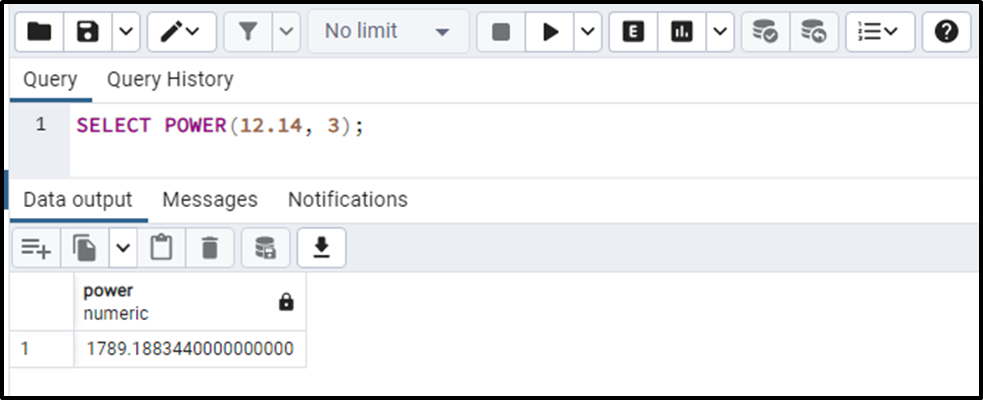The output shows that the POWER() function retrieves the accurate value.

Example 4: How to Use POWER() Function on Negative Values?

In this example, we will use all three possibilities to use a negative value in POWER function:

```SELECT POWER(-5, 5),
POWER(-5, -5),
POWER(5, -5);```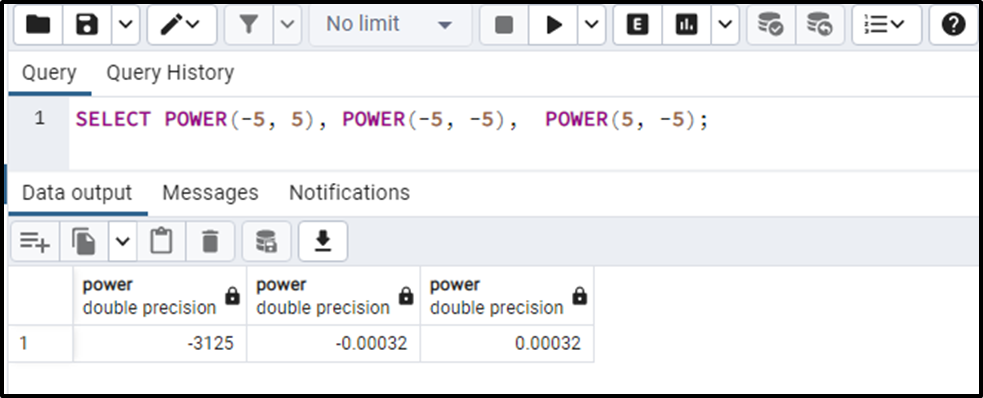This is how the POWER() function works on negative values.

Example 5: Calculate the Power of Large Values

Let’s execute the following command to see how the POWER() function works on large values:

`SELECT POWER(500, 12);`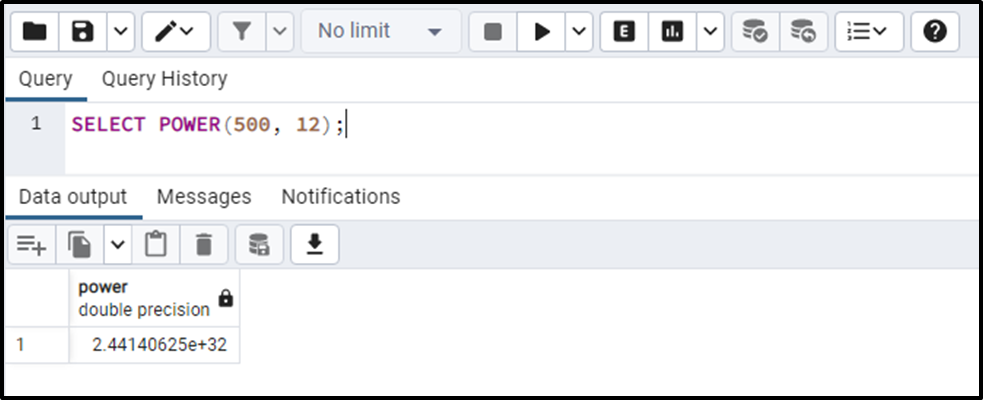This way, the POWER() function deals with the large values.

Example 6: How to Use POWER() Function on Table’s Data?

We have created a table named find_power, whose data is shown in the following snippet: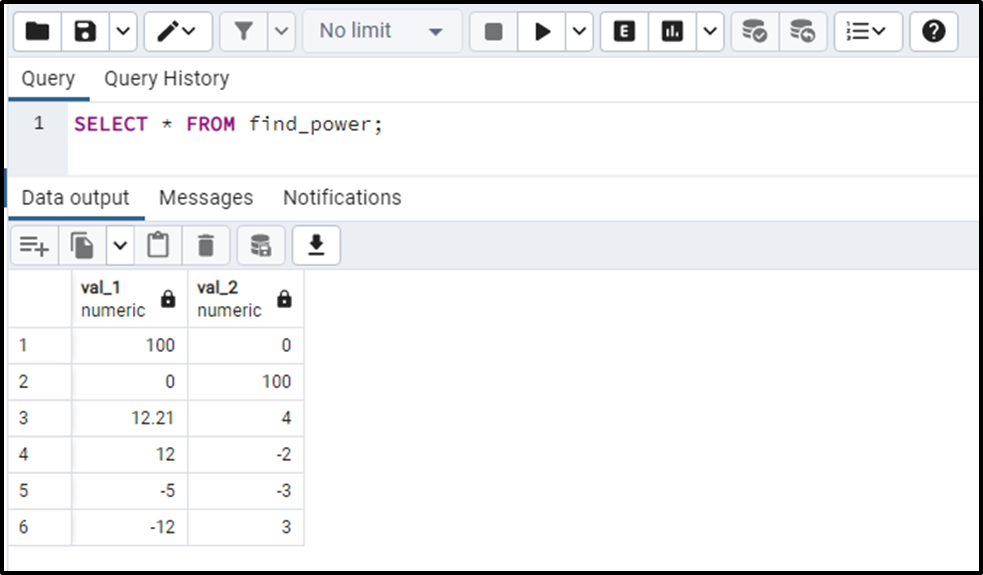The find_power table contains various values. The below-provided table will show you an in-depth understanding of the POWER() function:

```SELECT val_1, val_2, POWER(val_1, val_2)
FROM find_power;```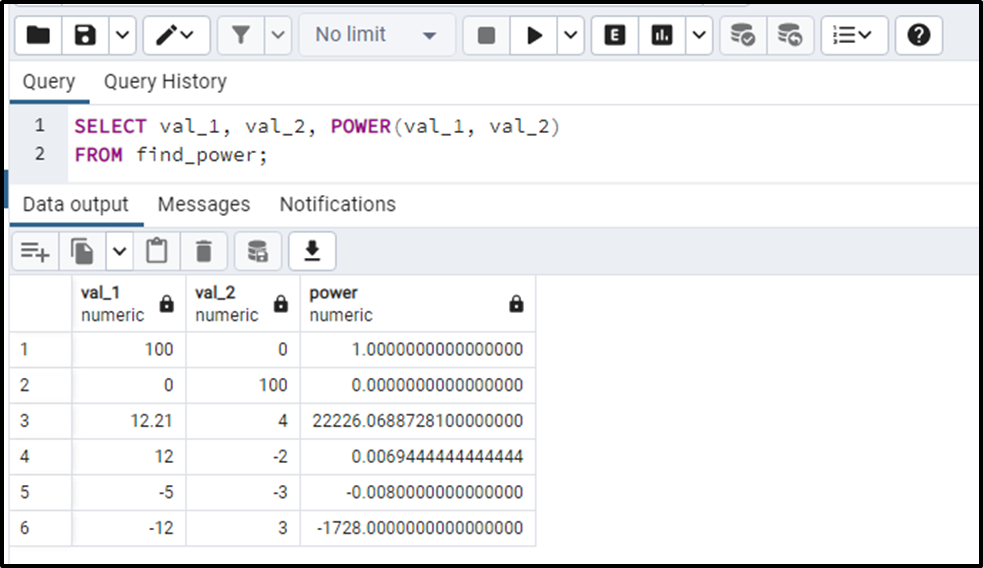This is how the POWER() function works on the table’s data.

Conclusion

The POWER() or POW() function accepts two numeric values as arguments and retrieves the first value/argument raised to the power of the second value/argument. The POWER() function can calculate the power of any number, i.e., positive, negative, fractional, etc. This write-up explains Postgres’ POWER() function with practical examples.